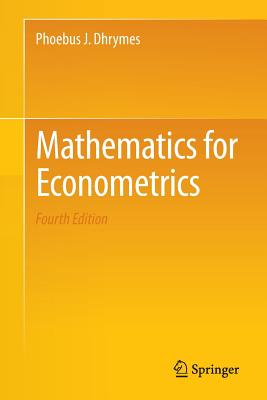### Mathematics for Econometrics

Paperback

List Price: 89.99*
* Individual store prices may vary.

#### Description

This book deals with a number of mathematical topics that are of great importance in the study of classical econometrics. There is a lengthy chapter on matrix algebra, which takes the reader from the most elementary aspects to the partitioned inverses, characteristic roots and vectors, symmetric, and orthogonal and positive (semi) definite matrices. The book also covers pseudo-inverses, solutions to systems of linear equations, solutions of vector difference equations with constant coefficients and random forcing functions, matrix differentiation, and permutation matrices. Its novel features include an introduction to asymptotic expansions, and examples of applications to the general-linear model (regression) and the general linear structural econometric model (simultaneous equations).

Springer, 9781461481447, 419pp.

Publication Date: September 24, 2013